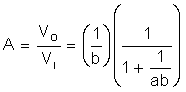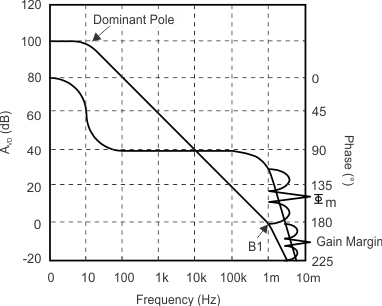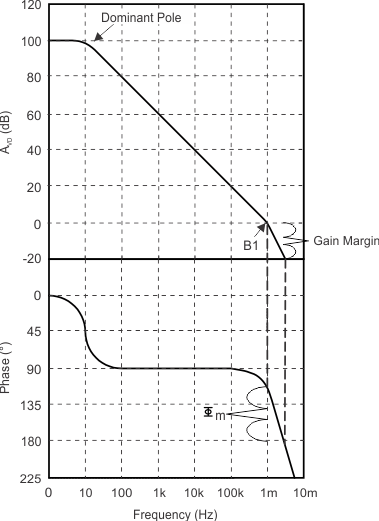SLOA011B January   2018  – July 2021

1. 1Introduction
2. 2Non-Inverting Amplifier
3. 3Inverting Amplifier
4. 4Simplified Op Amp Circuit Diagram
5. 5Op Amp Specifications
6. 6References
7. 7Glossary
8. 8Revision History

## 5.16 Unity-Gain Bandwidth and Phase Margin

There are five parameters that relate to the frequency characteristics of the op amp that you will encounter in Texas Instruments’ data sheets:

• Unity-gain bandwidth (B1)
• Gain bandwidth product (GBW)
• Phase margin at unity gain (φm)
• Gain margin
• Maximum output-swing bandwidth (BOM)

Unity-gain bandwidth (B1) and gain bandwidth product (GBW) are similar. B1 specifies the frequency at which AVD of the op amp is 1:

Equation 36. B1 = f @ AVD = 1

GBW specifies the gain-bandwidth product of the op amp in an open loop configuration and the output loaded:

Equation 37. GBW = AVD × f

Phase margin at unity gain (fm) is the difference between the amount of phase shift a signal experiences through the op amp at unity gain and 180°:

Equation 38. φm = 180° - phase shift @ B1

Gain margin is the difference between unity gain and the gain at 180° phase shift:

Equation 39. Gain margin = 1 – Gain @ 180° phase shift

Maximum output-swing bandwidth (BOM) specifies the bandwidth over which the output is above a specified value:

Equation 40. BOM = fMAX, while VO > VMIN

The limiting factor for BOM is slew rate. As the frequency gets higher and higher the output becomes slew rate limited and can not respond quickly enough to maintain the specified output voltage swing.

To make the op amp stable, capacitor, CC, is purposely fabricated on chip in the second stage (see Figure 4-1). This type of frequency compensation is termed dominant pole compensation. The idea is to cause the open-loop gain of the op amp to role off to unity before the output phase shifts by 180°. Remember that Figure 4-1 is very simplified: there are other frequency shaping elements within a real op amp. Figure 5-11 shows a typical gain vs. frequency plot for an internally compensated op amp as normally presented in a Texas Instruments data sheet. Figure 5-12 contains the same information except the phase axis is shifted for clarity.

As noted earlier, it can be seen that AVD falls off with frequency. AVD (and thus B1 or GBW) is a design issue when precise gain is required of a specific frequency band. Consider equation Equation 16, where the loop gain of the non-inverting amplifier is given by:

Equation 41.It is desired to control the gain of the circuit by selecting the appropriate resistors. The term 1/ab in the equation is seen as an error term. Unless a, or AVD, is large for all frequencies of interest in comparison with 1/b, a will have an effect on the gain of the circuit, which is undesired.

Phase margin (φm) and gain margin are different ways of specifying the stability of the circuit. Since rail-to-rail output op amps have higher output impedance, a significant phase shift is seen when driving capacitive loads. This extra phase shift erodes the phase margin, and for this reason most CMOS op amps with rail-to-rail outputs have limited ability to drive capacitive loads.Figure 5-11 Typical Large-Signal Differential Voltage Amplification and Phase Shift vs. FrequencyFigure 5-12 Easier to Read Graph of Voltage Amplification and Phase Shift vs. Frequency